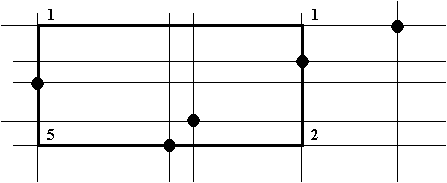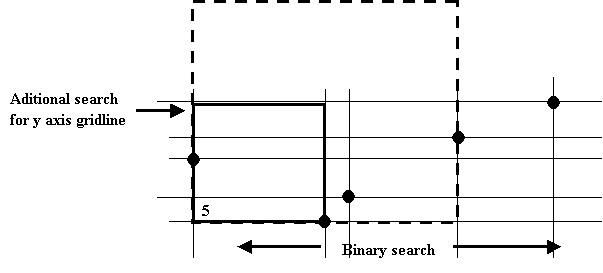# Internet Problem Solving Contest

## Solution to Problem G – Gardening

We denote |a|^ the smalest integer that is greater or equal to a.

Let's name the coordinates in the garden x and y. The x coordinate is increasing to the right and the y upwards. Let A be the square that is solving this problem. Let d be the length of the side of A. We will present the algorithm in several steps:

• We will specify additional conditions for the square that we search. These conditions will make the search less time consuming. We will proof that we can to that. That is: if there is a solution with the side of the square equal to d than there is a solution with the same square size where also the additional conditions hold. These conditions will be:
• (a) The square will contain exactly |n/2|^ plants.
• (b) The bottom-left corner of the square will have it's x and y coordinate identical with some of the plants (can be x coordinate of one plant and y of another).
• We will describe a algorithm that finds the square with minimal size that satisfies the conditions from the problem specification and the additional conditions mentioned above in O((n log n)2).

Theorem 1: We can assume that A satisfies (a) and (b).

Proof: Let's have a square B that solves the problem. It does contain at least |n/2|^ plants. By shifting this square up and to the right some plants will leave the area of the square and some will enter. As there are no plants with the same x or y coordinate only one plant can enter or leave the square at a time. If we shift the square upwards long enough eventually no plants will be contained inside. As the number of plants in the square changes only by one at a time there must be a shift s upwards where there are exactly |n/2|^ plants inside the square. Let m be the maximum shift upwards where this holds. If we shift square B by m than there will be a plant at the bottom side of the square (why? - easy contradiction with the "m is maximum" condition). We will name this square C. C has the y coordinate of it's bottom-left corner equal to the y coordinate of a plant.

Let's shift C to the right. Let n be the maximum shift to the right where the resulting square contains |n/2|^ plants. Than there will be a plant at the left side of the square. We will name this square D. D has the x coordinate of it's bottom-left corner equal to the x coordinate of a plant. The y coordinate of that corner did not change.

To any solution B we have found a solution D that satisfies the conditions of the theorem and therefore we can limit our search with that conditions as it is not our job to list all possible solutions.

Algorithm: Let's combine the x coordinate of all plants with all y coordinates. We will get n^2 points. Now let us count how many plants are to the top and to the left of each of these points (plants at the same level as the point are included). This can be done in O(n^2) (how?). Now it is relatively easy to count how many plants there are in any rectangle aligned with this grid. Just add the numbers that are in the bottom-left and top-right corners and subtract the numbers that are in the bottom-right and top-left corners. You have the number of plants inside the rectangle after doing some corrections for the plants that are on the edges (see picture - the number of plants inside the rectange is: 5+1-1-2 = 3, +1 plant at the right edge).By theorem 1. we assume that the bottom-left corner of the solving rectangle A is in one of the n^2 intersections. Let's find the minumun square with the bottom-left corner in each of these intersections that contains |n/2|^ plants. We will do this by doing a binary search for the size of the square. We will begin with some size of the square aligned with the grid on x axis. Than we will count the number of plants inside. To do this we have to know where does this square fit into the grid on the y axis (to do this we have to implement another binary search for the correct y axis gridline). If the resulting number of plants contained in the square is above the desired number than we will decrease the upper bound of the binary search. In the other case we will increase the lower bound of the binary search. After a limited number of steps we will arrive at a x axis gridline (if the number of contained plant does not equal to the desired number than we have to do some corrections along the y axis). This will specify a rectangle for arch of the n2 points. We will find the smallest of all this rectangles and by theorem 1. this rectangle has to be the solution of the problem.The time complexity of this algorithm is O((n log n)2).